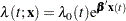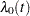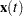The PHREG Procedure

The PHREG procedure fits the proportional hazards model of Cox (1972, 1975) to survival data that might be right censored. The Cox model is a semiparametric model in which the hazard function of the survival time is given bywhereis an unspecified baseline hazard function,is a vector of covariate values (possibly time-dependent), andis a vector of unknown regression parameters. The model is referred to as a semiparametric model because part of the model involves the unspecified baseline function over time (which has an infinite dimension) and the other part involves a finite number of regression parameters. Several texts that discuss the Cox regression models are Collett (1994), Cox and Oakes (1984), Kalbfleisch and Prentice (1980), and Lawless (1982). Extensions of the Cox model are discussed in Therneau and Grambsch (2000), Andersen et al. (1992), and Fleming and Harrington (1991). For more information about PROC PHREG, see Chapter 66, The PHREG Procedure.